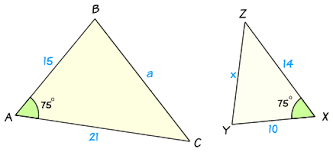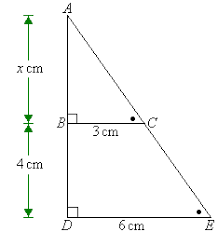Kindergarten
1st
2nd
3rd
4th
5th
6th
7th
8th
9th
10th
11th
12th
Higher Ed
Other
Subjects
ELA
Math
Science
Social Studies
Art
Computer Science
French
German
Music
Physical Education
Spanish
Other
Private Library
Similar Figures 1
starstarstarstarstarstarstarstarstarstar
by Jennifer Joyce
| 6 Questions
Note from the author:
Similar Figures1
1 pt
write a proportion demonstrating similarity betweent the triangles using only numbers2
1 pt
Write a proportion using only sides to demonstrate the similarity of the triangles. Use side AB in the proportion.3
1 pt
Write a proportion using angles to show similarity. Be sure to use <F in your proportion. You must also use the angle symbol in your proportion.4
1 pt
Which proportion correctly demonstrates similarity between the two triangles?
A

B

C

D5
1 pt
Which Proportion could be used to demonstrate similarity between the triangles using angles?
A

B

C

D6
1 pt
Which proportion could be used to calculate the lenfth of the missing side?
A

B

C

D

Add to my formatives list